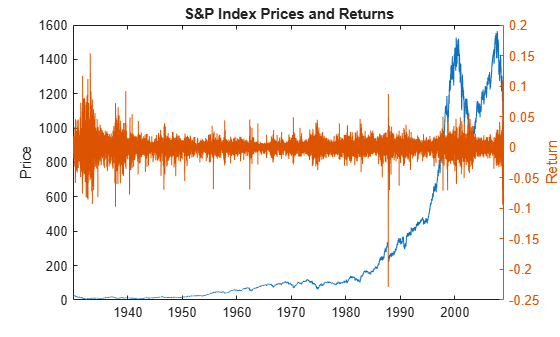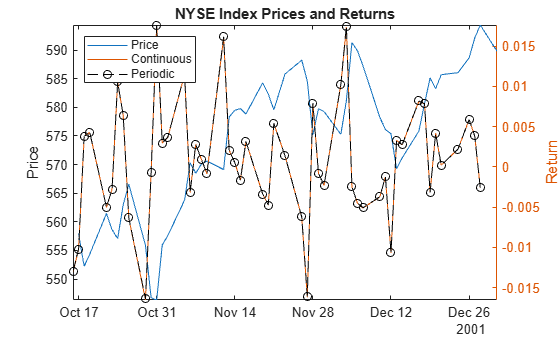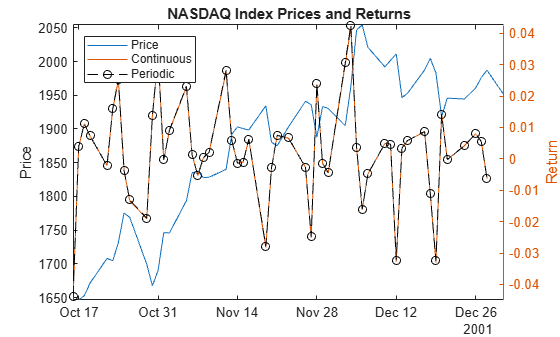# price2ret

Convert prices to returns

## Syntax

``[Returns,intervals] = price2ret(Prices)``
``ReturnTbl = price2ret(PriceTbl)``
``[___] = price2ret(___,Name=Value)``

## Description

example

````[Returns,intervals] = price2ret(Prices)` returns the matrix of `numVars` continuously compounded return series `Returns`, and corresponding time intervals `intervals`, from the matrix of `numVars` price series `Prices`.```

example

````ReturnTbl = price2ret(PriceTbl)` returns the table or timetable of continuously compounded return series `ReturnTbl` of each variable in the table or timetable of price series `PriceTbl`. To select different variables in `Tbl` from which to compute returns, use the `DataVariables` name-value argument.```

example

````[___] = price2ret(___,Name=Value)` uses additional options specified by one or more name-value arguments, using any input-argument combination in the previous syntaxes. `price2ret` returns the output-argument combination for the corresponding input arguments. For example, `price2ret(Tbl,Method="periodic",DataVariables=1:5)` computes the simple periodic returns of the first five variables in the input table `Tbl`.```

## Examples

collapse all

Load the Schwert Stock data st `Data_SchwertStock.mat`, which contains daily prices of the S&P index from 1930 through 2008, among other variables (enter `Description` for more details).

```load Data_SchwertStock numObs = height(DataTableDly)```
```numObs = 20838 ```
`dates = datetime(datesDly,ConvertFrom="datenum");`

Convert the S&P price series to returns.

```prices = DataTableDly.SP; returns = price2ret(prices);```

`returns` is a 20837-by-1 vector of daily S&P returns compounded continuously.

`r9 = returns(9)`
```r9 = 0.0033 ```
`p9_10 = [prices(9) prices(10)]`
```p9_10 = 1×2 21.4500 21.5200 ```

`returns(9) = 0.0033 `is the daily return of the prices in the interval [21.45, 21.52].

```plot(dates,DataTableDly.SP) ylabel("Price") yyaxis right plot(dates(1:end-1),returns) ylabel("Return") title("S&P Index Prices and Returns")```Convert the price series in a table to simple periodic return series.

Load the US equity indices data set, which contains the table `DataTable` of daily closing prices of the NYSE and NASDAQ composite indices from 1990 through 2011.

`load Data_EquityIdx`

Create a timetable from the table.

```dates = datetime(dates,ConvertFrom="datenum"); TT = table2timetable(DataTable,RowTimes=dates); numObs = height(TT);```

Convert the NASDAQ and NYSE prices to simple periodic and continuously compounded returns.

```varnames = ["NASDAQ" "NYSE"]; TTRetC = price2ret(TT,DataVariables=varnames); TTRetP = price2ret(TT,DataVariables=varnames,Method="periodic");```

Because `TT` is a timetable, `TTRetC` and `TTRetP` are timetables.

Plot the return series with the corresponding prices for the last 50 observations.

```idx = ((numObs - 1) - 51):(numObs - 1); figure plot(dates(idx + 1),TT.NYSE(idx + 1)) title("NYSE Index Prices and Returns") ylabel("Price") yyaxis right h = plot(dates(idx),[TTRetC.NYSE(idx) TTRetP.NYSE(idx)]); h(2).Marker = 'o'; h(2).Color = 'k'; ylabel("Return") legend(["Price" "Continuous" "Periodic"],Location="northwest") axis tight``````figure plot(dates(idx + 1),TT.NASDAQ(idx + 1)) title("NASDAQ Index Prices and Returns") ylabel("Price") yyaxis right h = plot(dates(idx),[TTRetC.NASDAQ(idx) TTRetP.NASDAQ(idx)]); h(2).Marker = 'o'; h(2).Color = 'k'; ylabel("Return") legend(["Price" "Continuous" "Periodic"],Location="northwest") axis tight```In this case, the simple periodic and continuously compounded returns of each price series are similar.

Create two stock price series from continuously compounded returns that have the following characteristics:

• Series 1 grows at a 10 percent rate at each observation time.

• Series 2 changes at a random uniform rate in the interval [-0.1, 0.1] at each observation time.

• Each series starts at price 100 and is 10 observations in length.

```rng(1); % For reproducibility numObs = 10; p1 = 100; r1 = 0.10; r2 = [0; unifrnd(-0.10,0.10,numObs - 1,1)]; s1 = 100*exp(r1*(0:(numObs - 1))'); cr2 = cumsum(r2); s2 = 100*exp(cr2); S = [s1 s2];```

Convert each price series to a return series, and return the observation intervals.

`[R,intervals] = price2ret(S);`

Prepend the return series so that the input and output elements are of the same length and correspond.

`[[NaN; intervals] S [[NaN NaN]; R] r2]`
```ans = 10×6 NaN 100.0000 100.0000 NaN NaN 0 1.0000 110.5171 98.3541 0.1000 -0.0166 -0.0166 1.0000 122.1403 102.7850 0.1000 0.0441 0.0441 1.0000 134.9859 93.0058 0.1000 -0.1000 -0.1000 1.0000 149.1825 89.4007 0.1000 -0.0395 -0.0395 1.0000 164.8721 83.3026 0.1000 -0.0706 -0.0706 1.0000 182.2119 76.7803 0.1000 -0.0815 -0.0815 1.0000 201.3753 72.1105 0.1000 -0.0627 -0.0627 1.0000 222.5541 69.9172 0.1000 -0.0309 -0.0309 1.0000 245.9603 68.4885 0.1000 -0.0206 -0.0206 ```

`price2ret` returns rates matching the rates from the simulated series. `price2ret` assumes prices are recorded in a regular time base. Therefore, all durations between prices are `1`.

Convert the prices to returns again, but associate the prices with years starting from August 1, 2010.

```tau1 = datetime(2010,08,01); dates = tau1 + years((0:(numObs-1))'); [Ry,intervalsy] = price2ret(S,Ticks=dates); [[NaN; intervalsy] S [[NaN NaN]; Ry] r2]```
```ans = 10×6 NaN 100.0000 100.0000 NaN NaN 0 365.2425 110.5171 98.3541 0.0003 -0.0000 -0.0166 365.2425 122.1403 102.7850 0.0003 0.0001 0.0441 365.2425 134.9859 93.0058 0.0003 -0.0003 -0.1000 365.2425 149.1825 89.4007 0.0003 -0.0001 -0.0395 365.2425 164.8721 83.3026 0.0003 -0.0002 -0.0706 365.2425 182.2119 76.7803 0.0003 -0.0002 -0.0815 365.2425 201.3753 72.1105 0.0003 -0.0002 -0.0627 365.2425 222.5541 69.9172 0.0003 -0.0001 -0.0309 365.2425 245.9603 68.4885 0.0003 -0.0001 -0.0206 ```

price2ret assumes time units are days. Therefore, all durations are approximately 365 and the returns are normalized for that time unit.

Compute returns again, but specify that the observation times are years.

```[Ryy,intervalsyy] = price2ret(S,Ticks=dates,Units="years"); [[NaN; intervalsyy] S [[NaN NaN]; Ryy] r2]```
```ans = 10×6 NaN 100.0000 100.0000 NaN NaN 0 1.0000 110.5171 98.3541 0.1000 -0.0166 -0.0166 1.0000 122.1403 102.7850 0.1000 0.0441 0.0441 1.0000 134.9859 93.0058 0.1000 -0.1000 -0.1000 1.0000 149.1825 89.4007 0.1000 -0.0395 -0.0395 1.0000 164.8721 83.3026 0.1000 -0.0706 -0.0706 1.0000 182.2119 76.7803 0.1000 -0.0815 -0.0815 1.0000 201.3753 72.1105 0.1000 -0.0627 -0.0627 1.0000 222.5541 69.9172 0.1000 -0.0309 -0.0309 1.0000 245.9603 68.4885 0.1000 -0.0206 -0.0206 ```

`price2ret` normalizes the returns relative to years, and now the returned rates match the simulated rates.

## Input Arguments

collapse all

Time series of prices, specified as a `numObs`-by-`numVars` numeric matrix. Each row of `Prices` corresponds to an observation time specified by the optional `Ticks` name-value argument. Each column of `Prices` corresponds to an individual price series.

Data Types: `double`

Time series of prices, specified as a table or timetable with `numObs` rows. Each row of `Tbl` is an observation time. For a table, the optional `Ticks` name-value argument specifies observation times. For a timetable, `PriceTbl.Time` specifies observation times and it must be a datetime vector.

Specify `numVars` variables, from which to compute returns, by using the `DataVariables` argument. The selected variables must be numeric.

### Name-Value Arguments

Specify optional pairs of arguments as `Name1=Value1,...,NameN=ValueN`, where `Name` is the argument name and `Value` is the corresponding value. Name-value arguments must appear after other arguments, but the order of the pairs does not matter.

Before R2021a, use commas to separate each name and value, and enclose `Name` in quotes.

Example: `price2ret(Tbl,Method="periodic",DataVariables=1:5)` computes the simple periodic returns of the first five variables in the input table `Tbl`.

Observation times τ, specified as a length `numObs` numeric or datetime vector of increasing values.

When the input price series are in a matrix or table, the default is `1:numObs`.

When the input price series are in a timetable, `price2ret` uses the row times in `PriceTbl.Time` and ignores `Ticks`. `PriceTbl.Time` must be a datetime vector.

Example: `Ticks=datetime(1950:2020,12,31)` specifies the end of each year from 1950 through 2020.

Example: `Ticks=datetime(1950,03,31):calquarters(1):datetime(2020,12,31)` specifies the end of each quarter during the years 1950 through 2020.

Data Types: `double` | `datetime`

Time units to use when observation times `Ticks` are datetimes, specified as a value in this table.

ValueDescription
`"milliseconds"`Milliseconds
`"seconds"`Seconds
`"minutes"`Minutes
`"hours"`Hours
`"days"`Days
`"years"`Years

`price2ret` requires time units to convert duration intervals to numeric values for normalizing returns.

When the value of the `Ticks` name-value argument is a numeric vector, `price2ret` ignores the value of `Units`.

Example: `Units="years"`

Data Types: `char` | `string`

Compounding method, specified as a value in this table.

ValueDescription
`"continuous"`Compute continuously compounded returns
`"periodic"`Compute simple periodic returns

Example: `Method="periodic"`

Data Types: `char` | `string`

Variables in `PriceTbl`, from which `price2ret` computes returns, specified as a string vector or cell vector of character vectors containing variable names in `PriceTbl.Properties.VariableNames`, or an integer or logical vector representing the indices of names. The selected variables must be numeric.

Example: `DataVariables=["GDP" "CPI"]`

Example: `DataVariables=[true true false false]` or `DataVariables=[1 2]` selects the first and second table variables.

Data Types: `double` | `logical` | `char` | `cell` | `string`

## Output Arguments

collapse all

Return series, returned as a (`numObs` – 1)-by-`numVars` numeric matrix. `price2ret` returns `Returns` when you supply the input `Prices`.

Returns in row i ri are associated with price interval [pi,pi+1], i = `1:(numObs - 1)`, according to the compounding method `Method`:

• When `Method` is `"continuous"`,

`${r}_{i}=\frac{\mathrm{log}\left({p}_{i+1}/{p}_{i}\right)}{{\tau }_{i+1}-{\tau }_{i}}.$`

• When `Method` is `"periodic"`,

`${r}_{i}=\frac{\left({p}_{i+1}/{p}_{i}\right)-1}{{\tau }_{i+1}-{\tau }_{i}}.$`

When observation times τ (see `Ticks`) are datetimes, the magnitude of the normalizing interval τi+1τi depends on the specified time units (see `Units`).

Time intervals between observations τi+1τi, returned as a length `numObs` – 1 numeric vector. `price2ret` returns `intervals` when you supply the input `Prices`.

When observation times (see `Ticks`) are datetimes, interval magnitudes depend on the specified time units (see `Units`).

Return series and time intervals, returned as a table or timetable, the same data type as `PriceTbl`, with `numObs` – 1 rows. `price2ret` returns `ReturnTbl` when you supply the input `PriceTbl`.

`ReturnTbl` contains the outputs `Returns` and `intervals`.

`ReturnTbl` associates observation time τi+1 with the end of the interval for the returns in row i ri.

## Algorithms

Consider the following variables:

The following figure shows how the inputs and outputs are associated.## Version History

Introduced before R2006a

expand all

Behavior changed in R2022a